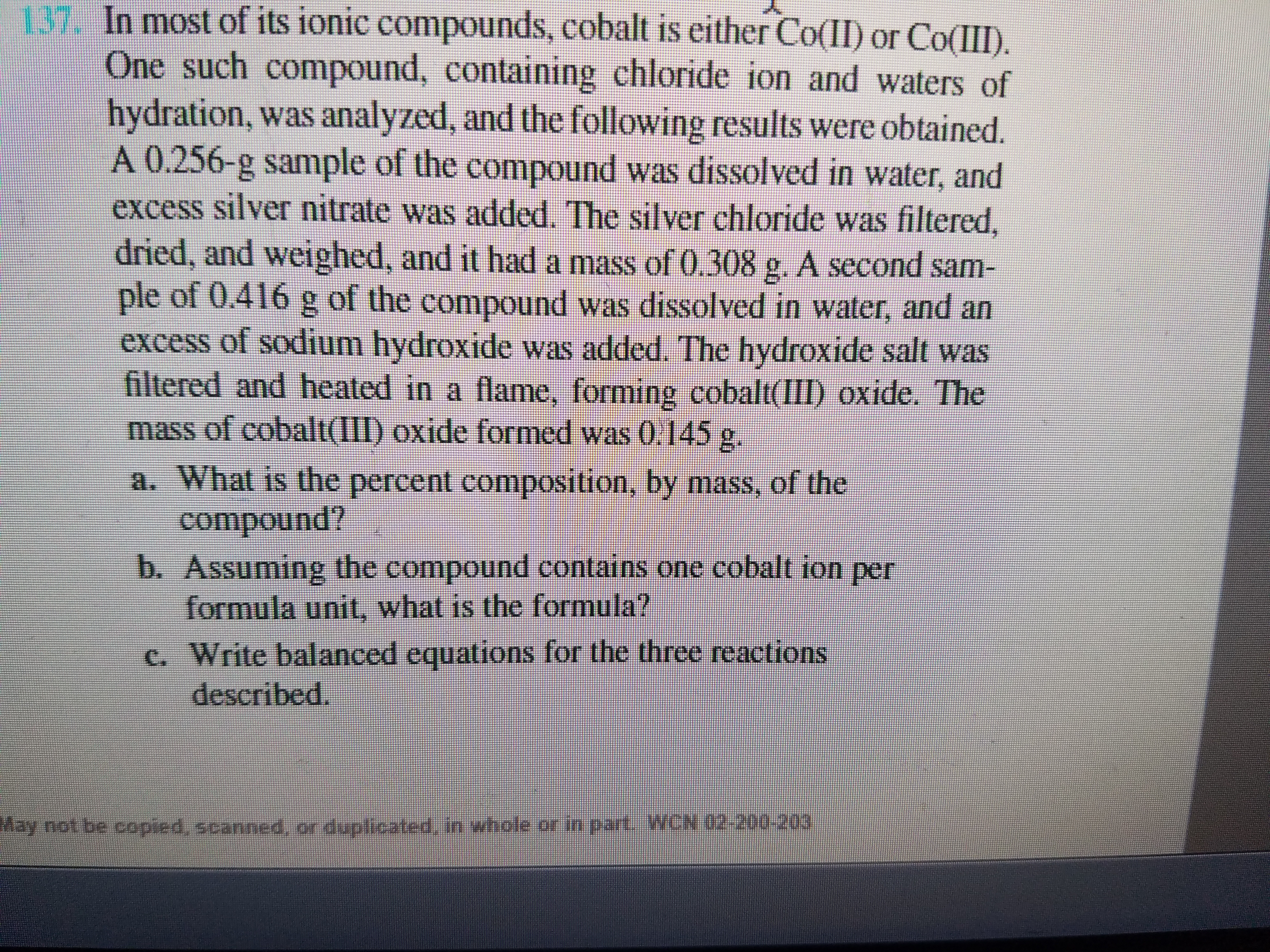# In most of ionic compounds, cobalt is either Co (II) or Co(III). One such compound, containing chloride ion and waters of hydration, was analyzed , and the following results were obtained. A 0.256- g sample of the compound was dissolved in water, and excess silver nitrate was added. The silver chloride was filtered, dried, and weighed, and it had a mass of .308 g. A second sample of .416 g of the compound was dissolved in water, and an excess of sodium hydroxide was added. The hydroxide salt was filtered and heated in a flame, forming cobalt (III) oxide. The mass of cobalt (III) oxide formed was 0.145 g. a) What is the percent composition, by mass, of the compound? b) Assuming the compound contains one cobalt atom per formula unit, what is the formula? c) Write balanced equations for the three reactions described.